## Integers

The term integers refers to all the whole numbers together with their opposites—not fractions or decimals.

For example, … –3, –2, –1, 0, 1, 2, 3, … are integers.

On a number line, numbers to the right of 0 are positive. Numbers to the left of 0 are negative, as shown in Figure 1.

This figure shows only the integers on the number line.

Given any two numbers on a number line, the one on the right is always larger, regardless of its sign (positive or negative).

When adding two integers with the same sign (either both positive or both negative), add the integers and keep the same sign.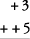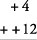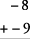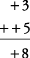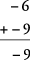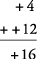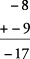When adding two integers with different signs (one positive and one negative), subtract the integers and keep the sign on the one with the larger value.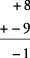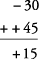Integers may also be added “horizontally.”

+8 + 11

–15 + 7

5 + (–3)

–21 + 6

+8 +11 = +19

–15 + 7 = –8

5 + (–3) = +2

–21 + 6 = –15

To subtract positive and/or negative integers, just change the sign of the number being subtracted and then use the rules for adding integers.

Subtract the following.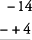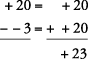Subtracting positive and/or negative integers may also be done “horizontally.”

Subtract the following.

+12 – (+4)

+16 – (–6)

–20 – (+3)

–5 – (–2)

+12 – (+4) = +12 + (–4) = 8

+16 – (–6) = +16 + (+6) = 22

–20 – (+3) = –20 + (–3) = –23

–5 – (–2) = –5 + (+2) = –3

If a minus precedes a parenthesis, it means that everything within the parentheses is to be subtracted. Therefore, using the same rule as in subtraction of integers, simply change every sign within the parentheses to its opposite and then add.

Subtract the following.

9 – (+3 – 5 + 7 – 6)

20 – (+35 – 50 + 100)

9 – (+3 – 5 + 7 – 6) = 9 + (–3 + 5 – 7 + 6)

= 9 + (+1)

= 10

20 – (+35 – 50 + 100) = 20 + (–35 + 50 – 100)

= 20 + (–85)

= –65

Or, if you can, total the numbers within the parentheses by first adding the positive numbers together, next adding the negative numbers together, then combining, and finally subtracting.

Subtract the following.

9 – (+3 – 5 + 7 – 6)

20 – (+35 – 50 + 100)

3 – (1 – 4)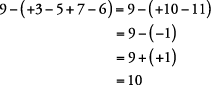Remember: If there is no sign given, the number is understood to be positive.orTo multiply or divide integers, treat them just like regular numbers but remember this rule: An odd number of negative signs produces a negative answer. An even number of negative signs produces a positive answer.

Multiply or divide the following.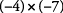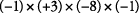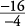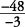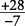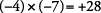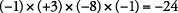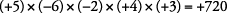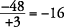The numerical value when direction or sign is not considered is called the absolute value. The absolute value of a number is written | |. Therefore, |3| = 3 and |–4 | = 4. The absolute value of a number is always positive except when the number is 0. The absolute value of zero is zero, | 0 | = 0.

Give the value.

|5|

| –8|

|3 – 9|

3 – | –6|

|5| = 5

|–8| = 8

|3 – 9| = |–6| = 6

3 – |–6| = 3 – 6 = –3

Note: Absolute value is taken first or work is done within absolute value brackets.